# MP Board Class 10th Maths Solutions Chapter 15 Probability Ex 15.1

In this article, we will share MP Board Class 10th Maths Book Solutions Chapter 15 Probability Ex 15.1 Pdf, These solutions are solved subject experts from the latest edition books.

## MP Board Class 10th Maths Solutions Chapter 15 Probability Ex 15.1

Question 1.
Complete the following statements:
(i) Probability of an event E + Probability of the event ‘not E’ = _________
(ii) The probability of an event that cannot happen is ______.Such an event is called _________.
(iii) The probability of an event that is certain to happen is ______ Such an event is called ______
(iv) The sum of the probabilities of all the elementary events of an experiment is ______.
(v) The probability of an event is greater than or equal to _______ and less than or equal to ______.
Solution:
(i) 1 : Probability of an event E + Probability of the event ‘not E’ = 1.
(ii) 0, impossible: The probability of an event that cannot happen is 0. Such an event is called impossible event.
(iii) 1, certain: The probability of an event that is certain to happen is 1. Such an event is called a sure or certain event.
(iv) 1: The sum of the probabilities of all the elementary events of an experiment is 1.
(v) 0, 1: The probability of an event is greater than or equal to 0 and less than or equal to 1.

Question 2.
Which of the following experiments have equally likely outcomes? Explain.
(i) A driver attempts to start a car. The car starts or does not start.
(ii) A player attempts to shoot a basketball. She/he shoots or misses the shot.
(iv) A baby is born. It is a boy or a girl.
Solution:
(i) It depends on various factors such as whether the car will start or not. So, the probability of car will start does not equal to the probability of car will not start.
∴ The outcomes are not equally likely.
(ii) It depends on the player’s ability. So, probability that the player shot the ball is not the same as the probability that the player misses the shot.
(iii) The outcomes are equally likely as the probability of answer either right or wrong is $$\frac{1}{2}$$
(iv) The outcomes are equally likely as the probability of ‘newly born baby to be either bay or girls’ is $$\frac{1}{2}$$ .

Question 3.
Why is tossing a coin considered to be a fair way of deciding which team should get the ball at the beginning of a football game?
Solution:
Since on tossing a coin, the outcomes ‘head’ and ‘tail’ are equally likely, the result of tossing a coin is completely unpredictable and so it is a fairway.Question 4.
Which of the following cannot be the probability of an event?
(A) $$\frac{2}{3}$$
(B) -1.5
(C) 15%
(D) 0.7
Solution:
Since, the probability of an event cannot be negative.
∴ -1.5 cannot be the probability of an event.

Question 5.
If P(E) = 0.05, what is the probability of ‘not E’?
Solution:
∵ P(E) + P(not E) = 1
∴ 0.05 + P(not E) = 1 ⇒ P(not E) = 0.95
Thus, probability of ‘not E’ = 0.95.

Question 6.
A bag contains lemon flavoured candies only. Malini takes out one candy without looking into the bag. What is the probability that she takes out
(i) an orange flavoured candy?
(ii) a lemon flavoured candy?
Solution:
(i) Since there are only lemon flavoured candies in the bag.
∴ Taking out orange flavoured candy is not possible.
⇒ Probability of taking out an orange flavoured candy = 0.

(ii) Probability of taking out a lemon flavoured candy = 1.

Question 7.
It is given that in a group of 3 students, the probability of 2 students not having the same birthday is 0.992. What is the probability that the 2 students have the same birthday?
Solution:
Let the probability of 2 students having same birthday = P(SB)
And the probability of 2 students not having the same birthday = P(NSB)
∴ P(SB) + P(NSB) = 1
⇒ P(SB) + 0.992 = 1 ⇒ P(SB) = 1 – 0.992 = 0.008

Question 8.
A bag contains 3 red balls and 5 black balls. A ball is drawn at random from the bag. What is the probability that the ball drawn is
(i) red?
(ii) not red?
Solution:
Total number of balls = 3 + 5 = 8
∴ umber of possible outcomes = 8
(i) ∵ There are 3 red balls.
∴ Number of favourable outcomes = 3
∴ P (red) = $$\frac{\text { Number of favourable outcomes }}{\text { Number of all possible outcomes }}$$
= $$\frac{3}{8}$$
(ii) Probability of the ball drawn which is not red = 1 – P(red) = $$1-\frac{3}{8}=\frac{8-3}{8}=\frac{5}{8}$$Question 9.
A box contains 5 red marbles, 8 white marbles and 4 green marbles. One marble is taken out of the box at random. What is the probability that the marble taken out will be
(i) red?
(ii) white?
(iii) not green?
Solution:
Total number of marbles = 5 + 8 + 4 = 17
∴ Number of all possible outcomes = 17
(i) ∵ Number of red marbles = 5
∴ Number of favourable outcomes = 5
∴ Probability of red marbles, P(red) = $$\frac{\text { Number of favourable outcomes }}{\text { Number of all possible outcomes }}=\frac{5}{17}$$

(ii) Number of white marbles = 8
∴ Probability of white marbles, P(white) = $$\frac{\text { Number of favourable outcomes }}{\text { Number of all possible outcomes }}=\frac{8}{17}$$
_ Number of favourable outcomes _ 8 Number of all possible outcomes 17

(iii) Number of green marbles = 4 Number of marbles which are not green
= 17-4 = 13
i.e., Favourable outcomes = 13
∴ Probability of marbles ‘not green’, P(not greeen)
$$\frac{\text { Number of favourable outcomes }}{\text { Number of all possible outcomes }}=\frac{13}{17}$$

Question 10.
A piggy bank contains hundred 50p coins, fifty ₹ 1 coins, twenty ₹ 2 coins and ten ₹ 5 coins. If it is equally likely that one of the coins will fall out when the bank is turned upside down, what is the probability that the coin
(i) will be a 50p coin?
(ii) will not be a ₹ 5 coin?
Solution:
Number of coins 50 p = 100, ₹ 1 = 50 ₹ 2 = 20, ₹ 5 = 10
Total number of coins = 100 + 50 + 20 +10 = 180
∴ Total possible outcomes = 180

(i) For a 50 p coin:
Favourable outcomes = 100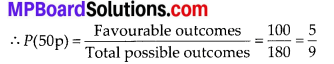(ii) For not a ₹ 5 coin:
Y Number of ₹ 5 coins = 10
∴ Number of ‘not ₹ 5’ coins = 180 – 10 = 170
⇒ Favourable outcomes = 170Question 11.
Gopi buys a fish from a shop for his aquarium. The shopkeeper takes out one fish at random from a tank containing 5 male fish and 8 female fish. What is the probability that the fish taken out is a male fish?
Solution:
Number of male fishes = 5
Number of female fishes = 8
∴ Total number of fishes = 5 + 8 = 13
⇒ Total number of outcomes = 13
For a male fish:
Number of favourable outcomes = 5Question 12.
A game of chance consists of spinning an arrow which comes to rest pointing at one of the numbers 1, 2, 3, 4, 5, 6, 7, 8 (see figure), and these are equally likely outcomes. What is the probability that it will point at
(i) 8?
(ii) an odd number?
(iii) a number greater than 2?
(iv) a number less than 9?Solution:
Total number marked = 8
∴ Total number of possible outcomes = 8
(i) When pointer points at 8:
Number of favourable outcomes = 1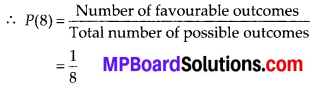(ii) When pointer points at an odd number:
∵ Odd numbers are 1, 3, 5 and 7
∴ Total odd numbers from 1 to 8 = 4
⇒ Number of favourable outcomes = 4(iii) When pointer points at a number greater than 2:
∵ The numbers 3, 4, 5, 6, 7 and 8 are greater than 2
∴ Total numbers greater than 2 = 6
⇒ Number of favourable outcomes = 6(iv) When pointer points at a number less than 9:
∵ The numbers 1, 2, 3, 4, 5, 6, 7 and 8 are less than 9.
∴ Total numbers less than 9 = 8
∴ Number of favourable outcomes = 8Question 13.
A die is thrown once. Find the probability of getting:
(i) a prime number;
(ii) a number lying between 2 and 6;
(iii) an odd number.
Solution:
Since, numbers on a die are 1, 2, 3, 4, 5 and 6.
∴ Total number of possible outcomes = 6
(i) Since 2, 3 and 5 are prime number.
∴ Favourable outcomes = 3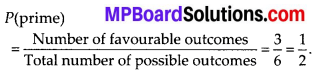(ii) Since the numbers between 2 and 6 are 3, 4 and 5
∴ Favourable outcomes = 3(iii) Since 1, 3 and 5 are odd numbers.
⇒ Favourable outcomes = 3Question 14.
One card is drawn from a well-shuffled deck of 52 cards. Find the probability of getting
(i) a king of red colour
(ii) a face card
(iii) a red face card
(iv) the jack of hearts
(vi) the queen of diamonds
Solution:
Number of cards in deck = 52
∴ Total number of possible outcomes = 52
(i) ∵ Number of red colour kings = 2
[∵ King of diamond and heart is red]
Number of favourable outcomes = 2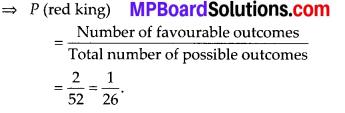(ii) For a face card:
∵ 4 kings, 4 queens and 4 jacks are face cards
∴ Number of face cards = 12
⇒ Number of favourable outcomes = 12(iii) Since, cards of diamond and heart are red
∴ There are 2 kings, 2 queens, 2 jacks i.e., 6 cards are red face cards.
∴ Number of favorable outcomes = 6(iv) Since, there is only 1 jack of hearts.
∴ Number of favourable outcomes = 1(v) There are 13 spades in a pack of 52 cards.
∴ Number of favourable outcomes = 13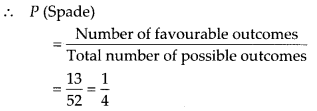(vi) ∵ There is only one queen of diamond.
∴ Number of favourable outcomes = 1Question 15.
Five cards-the ten, jack, queen, king and ace of diamonds, are well-shuffled with their face downwards. One card is then picked up at random.
(i) What is the probability that the card is the queen?
(ii) If the queen is drawn and put aside, what is the probability that the second card picked up is (a) an ace? (b) a queen?
Solution:
We have five cards.
∴ Total number of possible outcomes = 5
(i) ∵ Number of queen = 1
∴ Number of favourable outcomes = 1(ii) The queen is drawn and put aside.
∴ Only 5 – 1 = 4 cards are left.
∴ Total number of possible outcomes = 4
(a) ∵ There is only one ace.
∴ Number of favourable outcomes = 1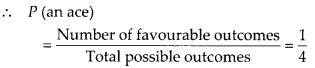(b) Since, the only queen has been put aside already.
∴ Number of favourable outcomes = 0Question 16.
12 defective pens are accidentally mixed with 132 good ones. It is not possible to just look at a pen and tell whether or not it is defective. One pen is taken out at random from this lot. Determine the probability that the pen taken out is a good one.
Solution:
We have number of good pens = 132 and number of defective pens = 12
∴ Total number of pens = 132 + 12 = 144 = Total possible outcomes
There are 132 good pens.
∴ Number of favourable outcomes = 132Question 17.
(i) A lot of 20 bulbs contain 4 defective ones. One bulb is drawn at random from the lot. What is the probability that this bulb is defective?
(ii) Suppose the bulb drawn in (i) is not defective and is not replaced. Now one bulb is drawn at random from the rest. What is the probability that this bulb is not defective?
Solution:
Since, there are 20 bulbs in the lot.
Total number of possible outcomes = 20
(i) ∵ Number of defective bulbs = 4
∴ Favourable outcomes = 4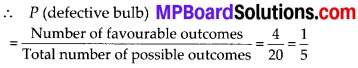(ii) ∵ The bulb drawn above is not included in the lot.
∴ Number of remaining bulbs = 20 – 1 = 19.
⇒ Total number of possible outcomes = 19.
∵ Number of bulbs which are not defective = 19 – 4 = 15
⇒ Number of favourable outcomes = 15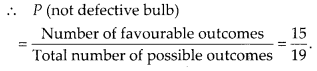Question 18.
A box contains 90 discs which are numbered from 1 to 90. If one disc is drawn at random from the box, find the probability that it bears
(i) a two-digit number
(ii) a perfect square number
(iii) a number divisible by 5.
Solution:
We have total number of discs = 90
∴ Total number of possible outcomes = 90
(i) Since the two-digit numbers are 10, 11, 12, ………, 90.
∴ Number of two-digit numbers = 90 – 9 = 81
∴ Number of favourable outcomes = 81(ii) Perfect square from 1 to 90 are 1, 4, 9, 16, 25, 36, 49, 64 and 81.
∴ Number of perfect squares = 9
∴ Number of favourable outcomes = 9(iii) Numbers divisible by 5 from 1 to 90 are 5, 10,15, 20, 25, 30, 35,40, 45, 50, 55, 60, 65, 70, 75, 80, 85, 90
i. e., There are 18 numbers from (1 to 90) which are divisible by 5.
∴ Numbers of favourable outcomes = 18Question 19.
A child has a die whose six faces show the letters as given below: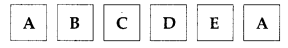The die is thrown once. What is the probability of getting (i) A? (ii) D?
Solution:
Since there are six faces of the given die and these faces are marked with letters∴ Total number of letters = 6
∴ Total number of possible outcomes = 6
(i) ∵ Number of faces having the letter A = 2
∴ Number of favourable outcomes = 2(ii) ∵ Number of faces having the letter D = 1
∴ Number of favourable outcomes = 1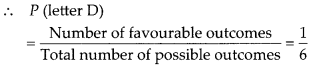Question 20.
20. Suppose you drop a die at random on the rectangular region shown in figure. What is the probability that it will land inside the circle with diameter 1 m?Solution:
Here, area of the rectangle = 3m × 2m = 6 m2
And, the area of the circle = πr2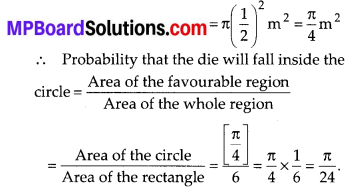Question 21.
A lot consists of 144 ball pens of which 20 are defective and the others are good. Nuri will buy a pen if it is good, but will not buy if it is defective. The shopkeeper draws one pen at random and gives it to her. What is the probability that
Solution:
Total number of ball pens = 144
⇒ Total number of possible outcomes = 144
(i) Since there are 20 defective pens.
∴ Number of good pens = 144 – 20 = 124
⇒ Number of favourable outcomes = 124(ii) Probability that Nuri will not buy it = 1 – [Probability that she will buy it]
= $$1-\frac{31}{36}=\frac{36-31}{36}=\frac{5}{36}$$

Question 22.
Two dice, one blue and one grey, are thrown at the same time. Write down all the possible outcomes. An event is defined as the sum of the two numbers appearing on the top of the dice.
(i) Complete the following table(ii) A student argues that’there are 11 possible outcomes 2, 3, 4, 5, 6, 7, 8, 9, 10, 11 and 12. Therefore, each of them has a probability $$\frac{1}{11}$$ Do you agree with this argument? Justify your answer.
Solution:
∵ The two dice are thrown together.
∴ Following are the possible outcomes :
(1, 1) ; (1, 2); (1, 3); (1, 4); (1, 5); (1, 6).
(2, 1) ; (2, 2); (2, 3); (2, 4); (2, 5); (2, 6).
(3, 1); (3, 2); (3, 3); (3, 4); (3, 5); (3, 6).
(4, 1) ; (4, 2); (4, 3); (4, 4); (4, 5); (4, 6).
(5, 1) ; (5, 2); (5, 3); (5, 4); (5, 5); (5, 6).
(6, 1) ; (6,.2); (6, 3); (6, 4); (6, 5); (6, 6).
∴ Total number of possible outcomes is 6 × 6 = 36
(i) (a) The sum on two dice is 3 for (1, 2) and (2, 1)
∴ Number of favourable outcomes = 2
⇒ P(3) = $$\frac{2}{36}$$

(b) The sum on two dice is 4 for (1, 3), (2, 2) and (3, 1).
∴ Number of favourable outcomes = 3
⇒ P(4) = $$\frac{3}{36}$$

(c) The sum on two dice is 5 for (1, 4), (2, 3), (3, 2) and (4,1)
∴ Number of favourable outcomes = 4
⇒ P(5) = $$\frac{5}{36}$$

(d) The sum on two dice is 6 for (1, 5), (2, 4), (3, 3), (4, 2) and (5,1)
∴ Number of favourable outcomes = 5
⇒ P(6) = $$\frac{5}{36}$$

(e) The sum on two dice is 7 for (1, 6), (2, 5), (3, 4), (4, 3), (5, 2) and (6,1)
∴ Number of favourable outcomes = 6
⇒ P(7) = $$\frac{62}{36}$$

(f) The sum on two dice is 9 for (3, 6), (4, 5), (5, 4) and (6, 3)
∴ Number of favourable outcomes = 4
⇒ P(9) = $$\frac{4}{36}$$

(g) The sum on two dice is 10 for (4, 6), (5, 5), (6,4)
∴ Number of favourable outcomes = 3
⇒ P(10) = $$\frac{3}{36}$$

(h) The sum on two dice is 11 for (5, 6) and (6,5)
∴ Number of favourable outcomes = 2
⇒ P(11) = $$\frac{2}{36}$$

Thus, the complete table is as follows:(ii) No. The number of all possible outcomes is 36 not 11.
∴ The argument is not correct.

Question 23.
A game consists of tossing a one rupee coin 3 times and noting its outcome each time. Hanif wins if all the tosses give the same result i.e., three heads or three tails, and loses otherwise. Calculate the probability that Hanif will lose the game.
Solution:
Let T denotes the tail and H denotes the head.
∴ All the possible outcomes are:
{H H H, H H T, H T T, T T T, T T H, T H T, T H H, H T H)
∴ Number of all possible outcomes = 8
Let the event that Hanif will lose the game denoted by E.
∴ Favourable events are: {HHT, HTH, THH, THT, TTH, HTT}
⇒ Number of favourable outcomes = 6
∴ P(E) = $$\frac{6}{8}=\frac{3}{4}$$

Question 24.
A die is thrown twice. What is the probability that
(i) 5 will not come up either time?
(ii) 5 will come up at least once?
[Hint: Throwing a die twice and throwing two dice simultaneously are treated as the same experiment]
Solution:
Since, throwing a die twice or throwing two dice simultaneously is the same.
∴ All possible outcomes are:
(1, 1); (1, 2); (1, 3); (1, 4); (1, 5); (1, 6).
(2, 1); (2, 2); (2, 3); (2, 4); (2, 5); (2, 6).
(3, 1) ; (3, 2); (3, 3); (3, 4); (3, 5); (3, 6).
(4, 1); (4, 2); (4, 3); (4, 4); (4, 5); (4, 6).
(5, 1) ; (5, 2); (5, 3); (5, 4); (5, 5); (5, 6).
(6, 1) ; (6, 2); (6, 3); (6, 4); (6, 5); (6, 6).
∴ All possible outcomes = 36
(i) Let E be the event that 5 does not come up either time.
∴ Numebr of favourable outcomes = [36 – (5 + 6)] = 25
∴ P(E) = $$\frac{25}{36}$$
(ii) Let N be the event that 5 will come up at least once, then number of favourable outcomes = 5 + 6 = 11
∴ P(N) = $$\frac{11}{36}$$Question 25.
Which of the following arguments are correct and which are not correct? Give reasons for your answer.
(i) If two coins are tossed simultaneously there are three possible outcomes – two heads, two tails or one of each. Therefore, for each of these outcomes, the probability is $$\frac{1}{3}$$.
(ii) If a die is thrown, there are two possible outcomes – an odd number or an even number. Therefore, the probability of getting an odd number is $$\frac{1}{3}$$
Solution:
(i) Given argument is not correct. Because, if two coins are tossed simultaneously then four outcomes are possible (HH, HT, TH, TT). So total outcomes is 4.
∴ The required probability = $$\frac{1}{4}$$.
(ii) Given argument is correct.
Since, total numebr of possible outcomes = 6
Odd numbers = 3 and even numbers = 3
So, favourable outcomes = 3 (in both the cases even or odd).
∴ Probability = $$\frac{3}{6}=\frac{1}{2}$$